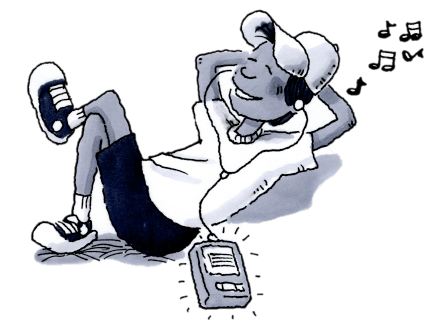### Home > CC2MN > Chapter 3 > Lesson 3.1.1 > Problem3-9

3-9.

Find the missing information from the following relationships.

1.Mark has downloaded four times as many songs on his music player as Chloe. If Mark has $440$ songs, how many songs does Chloe have?

Since Mark has $4$ times as many songs as Chloe and he has $440$ songs, what number of songs could Chloe have that would be a fourth of Mark's total songs?

$\text{Mark's songs}=4(\text{Chloe's songs})$

$440 \text{ songs} = 4(\text{Chloe's songs})$

What number times $4$ would equal $440$ songs? Use this equation to solve for Chloe's total number of songs.

2. Cici likes to collect shoes, but she only has half the number of pairs of shoes that her friend Aubree has. If Cici has $42$ pairs of shoes, how many pairs of shoes does Aubree have?

$\text{Cici's # of shoes }=\frac{1}{2}(\text{Aubree's # of shoes)}$

$2(\text{Cici's # of shoes})=\text{Aubree's # of shoes}$. Since Cici has $42$ pairs of shoes, use this equation to solve for the number of pairs of shoes Aubree has.

$84$ pairs of shoes.
Can you explain how you got this answer from the equation above?

3. Tito walked three more miles than Danielle. If Danielle walked $2$ miles, how far did Tito walk?

Refer to parts (a) and (b).

$\text{Tito's miles} = \text{Danielle's miles} + 3 \text{ miles}$